Function Repository Resource:

# SignedPermutations

Get all signed permutations of a list

Contributed by: Ed Pegg Jr and Jan Mangaldan
 ResourceFunction["SignedPermutations"][list] returns all the signed permutations of list. ResourceFunction["SignedPermutations"][list,spec] returns signed permutations of type spec.

## Details

Possible settings for spec include:
 All all permutations "Cyclic" cyclic permutations like {{0,1,2,3},{1,2,3,0},{2,3,0,1},{3,0,1,2}} "Even" permutations with an even number of transpositions "Odd" permutations with an odd number of transpositions "Symmetric" same as All

## Examples

### Basic Examples (1)

All signed permutations of the list {1,2,3}:

 In:=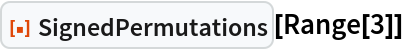Out=### Scope (2)

All signed even permutations of the list {1,2,3}:

 In:=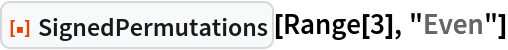Out=All signed cyclic permutations of the list {1,2,3}:

 In:=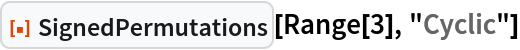Out=### Applications (2)

Find the vertices of a rhombic dodecahedron with edge lengths of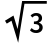:

 In:=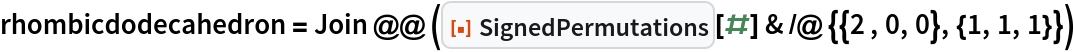Out=Use ConvexHullMesh to generate the rhombic dodecahedron from its vertices:

 In:=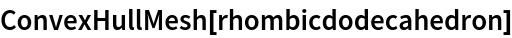Out=### Properties and Relations (2)

SignedPermutations[list,All] and SignedPermutations[list,"Symmetric"] are both equivalent to SignedPermutations[list]:

 In:=Out=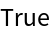Count all signed permutations corresponding to a given list, and compare with the expressions for the explicit counts:

 In:=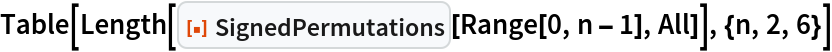Out=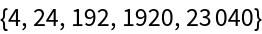In:=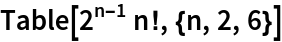Out=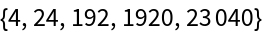In:=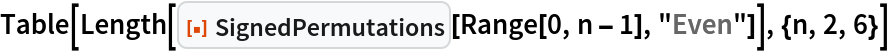Out=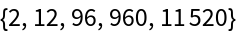In:=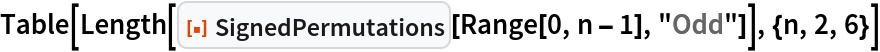Out=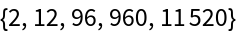In:=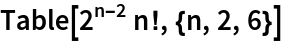Out=In:=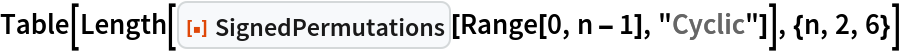Out=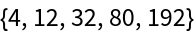In:=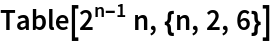Out=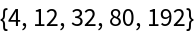### Possible Issues (2)

Create the 120 unit icosians that make the vertices of the 600-cell:

 In:=Out=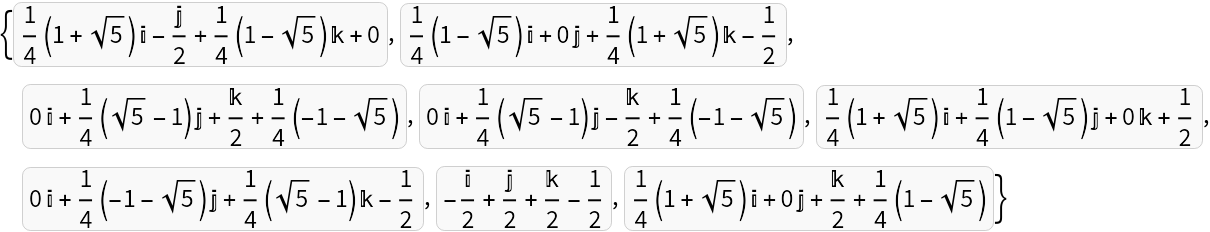The icosians are a closed group under NonCommutativeMultiply (**), but RootReduce was needed for predictable results:

 In:=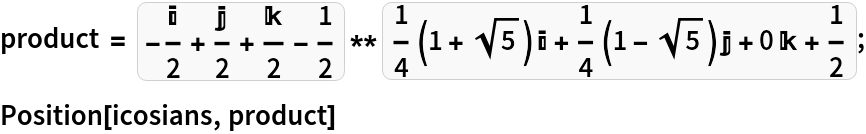Out=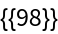### Neat Examples (3)

Create vertex sets for all 5 Platonic solids and 13 Archimedean solids with unit edges:

 In:=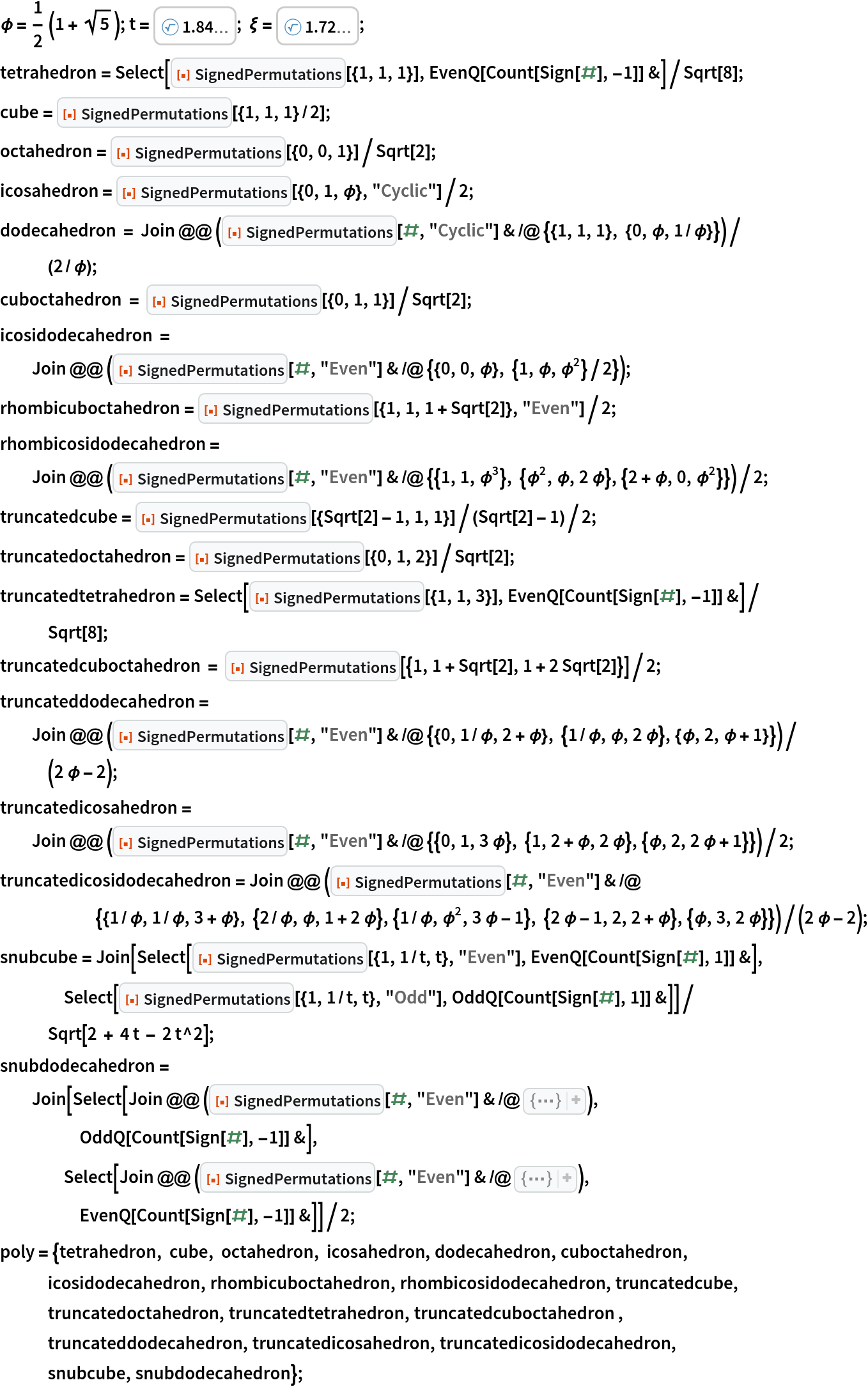Show these sets with a half-unit sphere around each vertex:

 In:=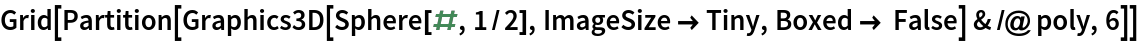Out=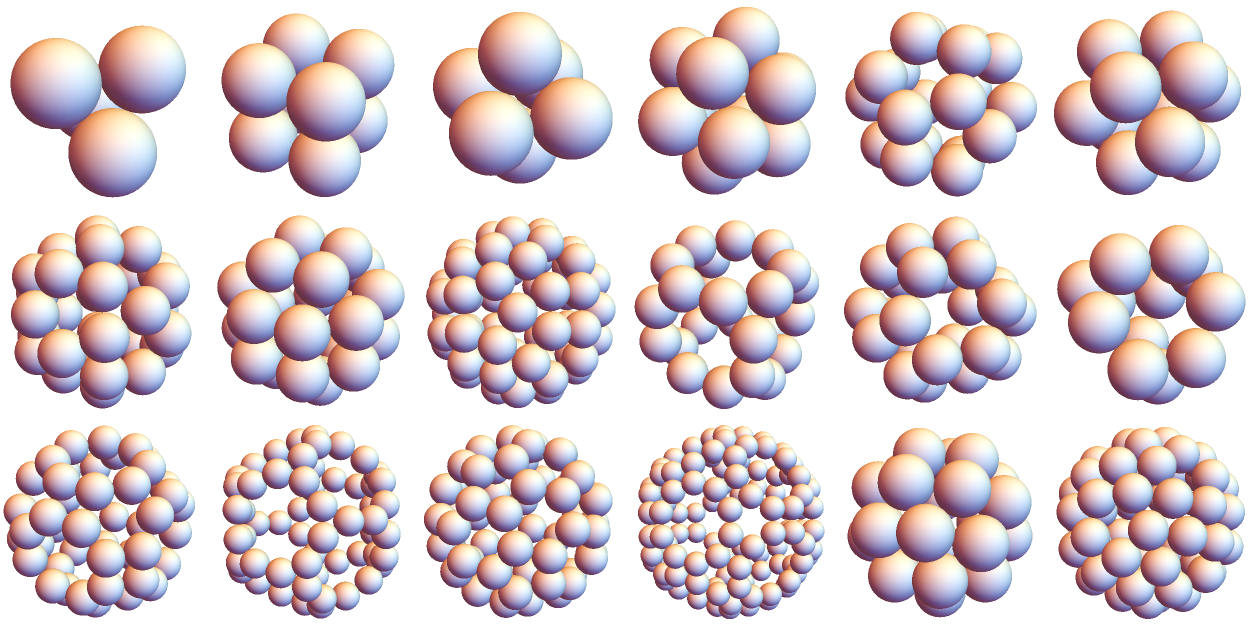Show the polyhedra:

 In:=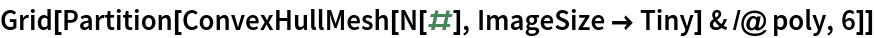Out=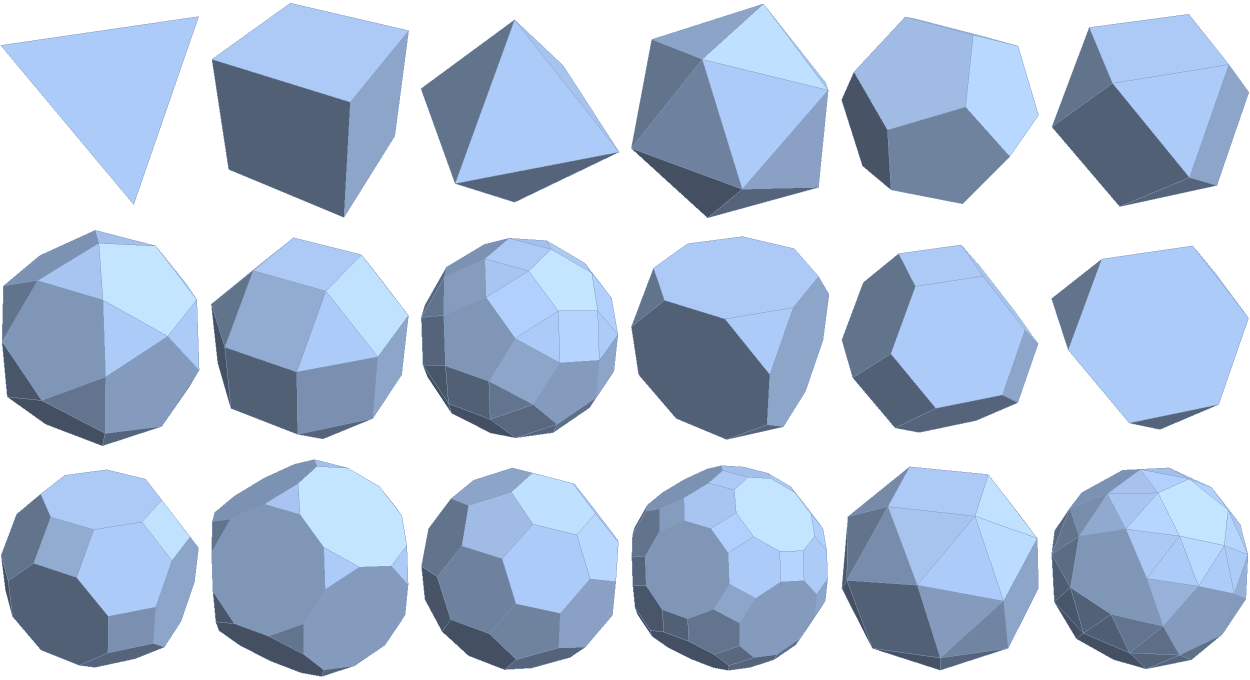## Version History

• 1.0.0 – 08 August 2022